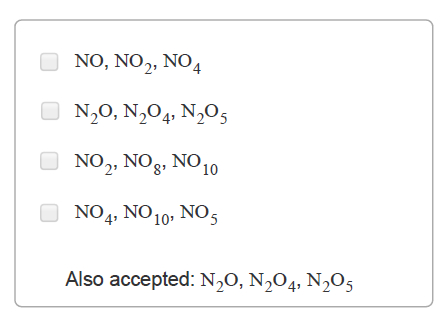# Problem: Part B. Samples of three different compounds were analyzed and the masses of each element were determined. CompoundMass NMass OA5.63.2B3.58.0C1.44.0If you were John Dalton and had never heard of a mole, which of the following would you think were possible sets of formulas for the compounds A, B, and C, respectively?Check all that apply.The law of multiple proportions predates the concept of a mole or molar mass. Thus, scientists like John Dalton could only determine the relative amounts of each type of atom in a formula, and not the exact formula. For example, Dalton could determine that there were two carbon-oxygen compounds, one with twice as much oxygen as the other. However, he did not know for sure that the formulas were CO and CO2. Other possibilities (given what they knew at the time) could have been C2O and C2O2, or CO2 and CO4, etc.

###### FREE Expert Solution
83% (84 ratings)View Complete Written Solution
###### Problem Details

Part B. Samples of three different compounds were analyzed and the masses of each element were determined.

 Compound Mass N Mass O A 5.6 3.2 B 3.5 8.0 C 1.4 4.0

If you were John Dalton and had never heard of a mole, which of the following would you think were possible sets of formulas for the compounds A, B, and C, respectively?

Check all that apply.The law of multiple proportions predates the concept of a mole or molar mass. Thus, scientists like John Dalton could only determine the relative amounts of each type of atom in a formula, and not the exact formula. For example, Dalton could determine that there were two carbon-oxygen compounds, one with twice as much oxygen as the other. However, he did not know for sure that the formulas were CO and CO2. Other possibilities (given what they knew at the time) could have been C2O and C2O2, or CO2 and CO4, etc.